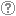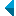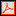# Iskanje po repozitorijuIskalni niz: išči po NaslovAvtorOpisKljučne besedeCelotno besediloLeto izida INALIIN NE išči po NaslovAvtorOpisKljučne besedeCelotno besediloLeto izida INALIIN NE išči po NaslovAvtorOpisKljučne besedeCelotno besediloLeto izida INALIIN NE išči po NaslovAvtorOpisKljučne besedeCelotno besediloLeto izida Vrsta gradiva: Vse vrste gradiv Habilitacijsko delo (m4) Višješolska diplomska naloga (m6) Specialistično delo (m3) Diplomsko delo * (dip) Magistrsko delo * (mag) Doktorska disertacija * (dok) Raziskovalni podatki ali korpus (data) * po starem in bolonjskem študiju Jezik: Vsi jezikiSlovenski jezikAngleški jezikNemški jezikHrvaški jezikSrbski jezikAfrikanski jezikAlbanski jezikArabski jezikArmenski jezikAzerbajdžanski jezikBolgarski jezikBosanski jezikČeški jezikČrnogorski jezikDanski jezikEstonski jezikFinski jezikFrancoski jezikGrški jezikGruzinski jezikIrski jezikItalijanski jezikJaponski jezikKatalonski jezikKazaški jezikKitajski jezikKorejski jezikLatinski jezikLatvijski jezikLitvanski jezikMadžarski jezikMakedonski jezikMalteški jezikNemški jezik (Avstrija)Nizozemski jezikNorveški jezikPoljski jezikPortugalski jezikRomunski jezikRuski jezikSlovanski jezik (ostali)Slovaški jezikSrbski jezik (cirilica)Španski jezikŠvedski jezikTurški jezikUkrajinski jezikNi določenVečjezičniNeznan jezikOstali Išči po: RUP    FAMNIT - Fakulteta za matematiko, naravoslovje in informacijske tehnologije    FHŠ - Fakulteta za humanistične študije    FM - Fakulteta za management    FTŠ Turistica - Fakulteta za turistične študije - Turistica    FVZ - Fakulteta za vede o zdravju    IAM - Inštitut Andrej Marušič    PEF - Pedagoška fakulteta    UPR - Univerza na Primorskem    ZUP - Založba Univerze na PrimorskemCOBISS    Univerza na Primorskem, Univerzitetna knjižnica - vsi oddelki Opcije: Prikaži samo zadetke s celotnim besedilom Ponastavi

 81 - 90 / 49756789101112131481.Testing whether the lifted group splitsRok Požar, 2016, izvirni znanstveni članekOpis: Let a group of automorphisms lift along a regular covering projection of connected graphs given combinatorially by means of voltages. The data that determine the lifted group and its action are then conveniently encoded in terms of voltages as well. Along these lines, an algorithm for testing whether the lifted group is a split extension of the group of covering transformations has recently been proposed in the case when the group of covering transformations is solvable. It consists of decomposing the covering into a series of coverings with elementary abelian groups of covering transformations, and inductively solving the problem at every elementary abelian step. Although the explicit construction of the lifted group is not needed, it still involves time and space consuming constructions of certain subgroups in the lifted group at every step except at the final one. In this paper, an improved version that completely avoids such constructions is presented. From voltage distribution we first compute the weak action and the factor set that determine the lifted group, and we then carry out the test by extracting the necessary information only from the corresponding weak actions and factor sets at every step. An experimental comparison is made against the previous version.Ključne besede: algorithm, graph, group extension, lifting automorphisms, regular covering projection, voltagesObjavljeno v RUP: 03.01.2022; Ogledov: 513; Prenosov: 17Celotno besedilo (317,95 KB) 82.On minimal forbidden subgraphs for the class of EDM-graphsGašper Jaklič, Jolanda Modic, 2015, izvirni znanstveni članekOpis: In this paper, a relation between graph distance matrices and Euclidean distance matrices (EDM) is considered. Graphs, for which the distance matrix is not an EDM (NEDM-graphs), are studied. All simple connected non-isomorphic graphs on ▫$n \le 8$▫ nodes are analysed and a characterization of the smallest NEDM-graphs, i.e., the minimal forbidden subgraphs, is given. It is proven that bipartite graphs and some subdivisions of the smallest NEDM-graphs are NEDM-graphs, too.Ključne besede: graph theory, graph, Euclidean distance matrix, distance, eigenvalueObjavljeno v RUP: 31.12.2021; Ogledov: 667; Prenosov: 15Celotno besedilo (711,65 KB) 83.The automorphism groups of non-edge transitive rose window graphsEdward Dobson, István Kovács, Štefko Miklavič, 2015, izvirni znanstveni članekOpis: In this paper, we determine the full automorphism groups of rose window graphs that are not edge-transitive. As the full automorphism groups of edge-transitive rose window graphs have been determined, this complete the problem of calculating the full automorphism group of rose window graphs. As a corollary, we determine which rose window graphs are vertex-transitive. Finally, we determine the isomorphism classes of non-edge-transitive rose window graphs.Ključne besede: rose window graphs, automorphism group, isomorphism problem, vertex-transitive graphObjavljeno v RUP: 31.12.2021; Ogledov: 641; Prenosov: 18Celotno besedilo (275,74 KB) 84.Some recent discoveries about half-arc-transitive graphs : dedicated to Dragan Marušič on the occasion of his 60th birthdayMarston D. E. Conder, Primož Potočnik, Primož Šparl, 2015, izvirni znanstveni članekOpis: We present some new discoveries about graphs that are half-arc-transitive (that is, vertex- and edge-transitive but not arc-transitive). These include the recent discovery of the smallest half-arc-transitive 4-valent graph with vertex-stabiliser of order 4, and the smallest with vertex-stabiliser of order 8, two new half-arc-transitive 4-valent graphs with dihedral vertex-stabiliser ▫$D_4$▫ (of order 8), and the first known half-arc-transitive 4-valent graph with vertex-stabiliser of order 16 that is neither abelian nor dihedral. We also use half-arc-transitive group actions to provide an answer to a recent question of Delorme about 2-arc-transitive digraphs that are not isomorphic to their reverse.Ključne besede: graph, edge-transitive, vertex-transitive, arc-transitive, half arc-transitiveObjavljeno v RUP: 31.12.2021; Ogledov: 552; Prenosov: 16Celotno besedilo (333,06 KB) 85.A census of 4-valent half-arc-transitive graphs and arc-transitive digraphs of valence two : dedicated to Dragan Marušič on the occasion of his 60th birthdayPrimož Potočnik, Pablo Spiga, Gabriel Verret, 2015, izvirni znanstveni članekOpis: A complete list of all connected arc-transitive asymmetric digraphs of in-valence and out-valence 2 on up to 1000 vertices is presented. As a byproduct, a complete list of all connected 4-valent graphs admitting a half-arc-transitive group of automorphisms on up to 1000 vertices is obtained. Several graph-theoretical properties of the elements of our census are calculated and discussed.Ključne besede: graphs, digraphs, edge-transitive, vertex-transitive, arc-transitive, half arc-transitiveObjavljeno v RUP: 31.12.2021; Ogledov: 601; Prenosov: 16Celotno besedilo (370,47 KB) 86.Reachability relations, transitive digraphs and groupsAleksander Malnič, Primož Potočnik, Norbert Seifter, Primož Šparl, 2015, izvirni znanstveni članekOpis: In [A. Malnič, D. Marušič, N. Seifter, P. Šparl and B. Zgrablič, Reachability relations in digraphs, Europ. J. Combin. 29 (2008), 1566-1581] it was shown that properties of digraphs such as growth, property ▫$\mathbf{Z}$▫, and number of ends are reflected by the properties of certain reachability relations defined on the vertices of the corresponding digraphs. In this paper we study these relations in connection with certain properties of automorphism groups of transitive digraphs. In particular, one of the main results shows that if atransitive digraph admits a nilpotent subgroup of automorphisms with finitely many orbits, then its nilpotency class and the number of orbits are closely related to particular properties of reachability relations defined on the digraphs in question. The obtained results have interesting implications for Cayley digraphs of certain types of groups such as torsion-free groups of polynomial growth.Ključne besede: Cayley digraph, reachability relationObjavljeno v RUP: 31.12.2021; Ogledov: 591; Prenosov: 16Celotno besedilo (311,92 KB) 87.Commuting graphs and extremal centralizersGregor Dolinar, Aleksandr Èmilevič Guterman, Bojan Kuzma, Polona Oblak, 2014, izvirni znanstveni članekOpis: We determine the conditions for matrix centralizers which can guarantee the connectedness of the commuting graph for the full matrix algebra ▫$M_n(\mathbb{F})$▫ over an arbitrary field ▫$\mathbb{F}$▫. It is known that if ▫$\mathbb{F}$▫ is an algebraically closed field and ▫$n \ge 3$▫, then the diameter of the commuting graph of ▫$M_n(\mathbb{F})$▫ is always equal to four. We construct a concrete example showing that if ▫$\mathbb{F}$▫ is not algebraically closed, then the commuting graph of ▫$M_n(\mathbb{F})$▫ can be connected with the diameter at least five.Ključne besede: commuting graph, matrix ring, centralizerObjavljeno v RUP: 31.12.2021; Ogledov: 533; Prenosov: 21Celotno besedilo (228,78 KB) 88.Sectional split extensions arising from lifts of groupsRok Požar, 2013, izvirni znanstveni članekOpis: Covering techniques have recently emerged as an effective tool used for classification of several infinite families of connected symmetric graphs. One commonly encountered technique is based on the concept of lifting groups of automorphisms along regular covering projections ▫$\wp \colon \tilde{X} \to X$▫. Efficient computational methods are known for regular covers with cyclic or elementary abelian group of covering transformations CT▫$(\wp)$▫. In this paper we consider the lifting problem with an additional condition on how a group should lift: given a connected graph ▫$X$▫ and a group ▫$G$▫ of its automorphisms, find all connected regular covering projections ▫$\wp \colon \tilde{X} \to X$▫ along which ▫$G$▫ lifts as a sectional split extension. By this we mean that there exists a complement ▫$\overline{G}$▫ of CT▫$(\wp)$▫ within the lifted group ▫$\tilde{G}$▫ such that ▫$\overline{G}$▫ has an orbit intersecting each fibre in at most one vertex. As an application, all connected elementary abelian regular coverings of the complete graph ▫$K_4$▫ along which a cyclic group of order 4 lifts as a sectional split extension are constructed.Ključne besede: covering projection, graph, group extension, lifting automorphisms, voltage assignmentObjavljeno v RUP: 31.12.2021; Ogledov: 633; Prenosov: 3Celotno besedilo (365,16 KB) 89.A note on homomorphisms of matrix semigroupMatjaž Omladič, Bojan Kuzma, 2013, izvirni znanstveni članekOpis: Let ▫$\mathbb{F}$▫ be a field. We classify multiplicative maps from ▫${\mathcal M}_n(\mathbb{F})$▫ to ▫${\mathcal M}_{n \choose k}(\mathbb{F})$▫ which annihilate a zero matrix and map rank-▫$k$▫ matrix into a rank-one matrix.Ključne besede: matrix semigroup, homomorphism, representationObjavljeno v RUP: 31.12.2021; Ogledov: 523; Prenosov: 17Celotno besedilo (247,09 KB) 90.Edge-contributions of some topological indices and arboreality of molecular graphsTomaž Pisanski, Janez Žerovnik, 2009, izvirni znanstveni članekOpis: Some graph invariants can be computed by summing certain values, called edge-contributions over all edges of graphs. In this note we use edge-contributions to study relationships among three graph invariants, also known as topological indices in mathematical chemistry: Wiener index, Szeged index and recently introduced revised Szeged index. We also use the quotient between the Wiener index and the revised Szeged index to study tree-likeness of graphs.Ključne besede: mathematical chemistry, chemical graph theory, topological index, revised Szeged indexObjavljeno v RUP: 30.12.2021; Ogledov: 520; Prenosov: 17Celotno besedilo (158,93 KB)
Iskanje izvedeno v 0.16 sek.
Na vrh